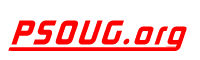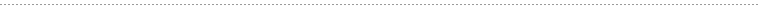Quick Search:Snippet Name: BIN_TO_NUM

Description: BIN_TO_NUM converts a bit vector to its equivalent number. Each argument to this function represents a bit in the bit vector. This function takes as arguments any numeric datatype, or any nonnumeric datatype that can be implicitly converted to NUMBER. Each expr must evaluate to 0 or 1. This function returns Oracle NUMBER.

BIN_TO_NUM is useful in data warehousing applications for selecting groups of interest from a materialized view using grouping sets.

Also see:

Comment: (none)

Language: PL/SQL
Highlight Mode: PLSQL

```BIN_TO_NUM(<value>,<value>,....)

-- The following example converts a binary value to a number:

SELECT BIN_TO_NUM(1,0,1,0) FROM DUAL;

BIN_TO_NUM(1,0,1,0)
-------------------
10
```